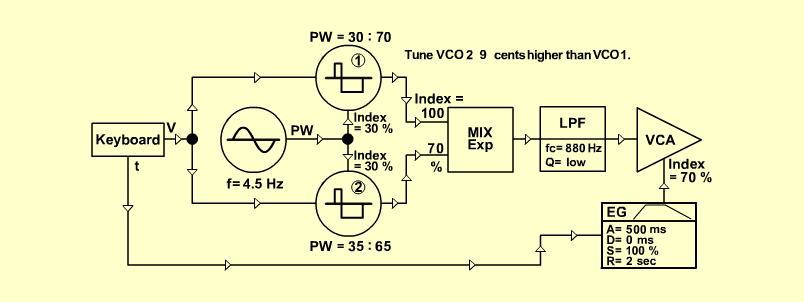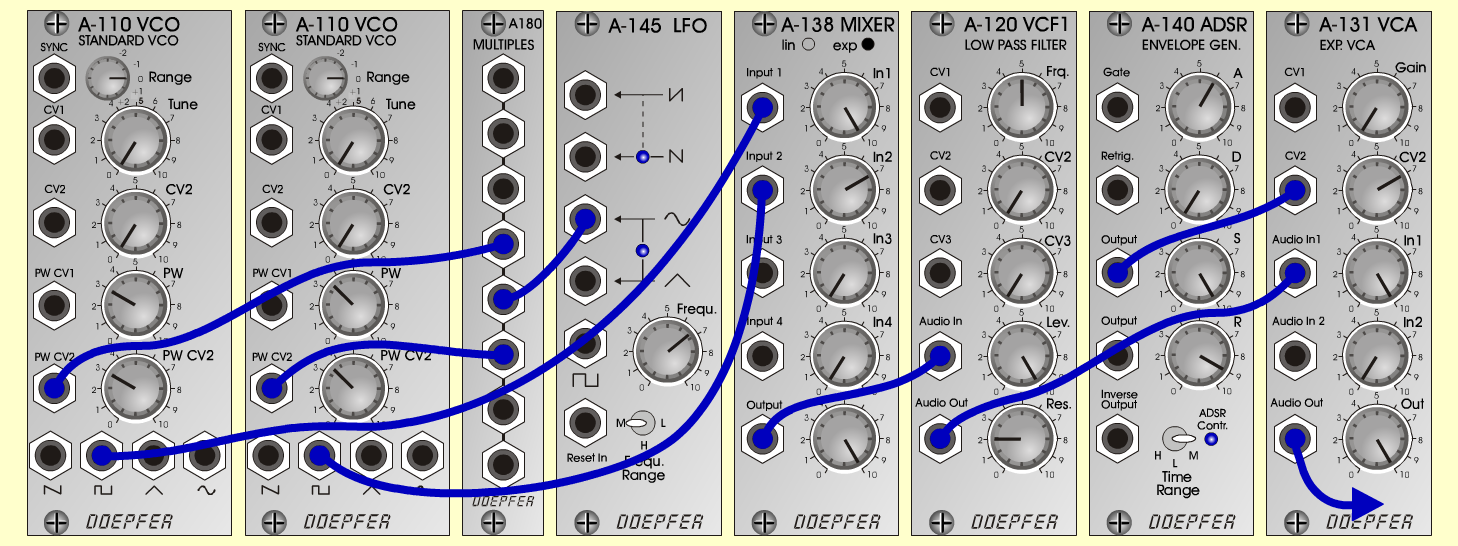MoviePadSynth- P a dD o e p f e r A - 100 Connections: Settings: A-145 (Sine)  <=>  A-180   A-180  <=>  A-110/1 (PW CV 2)   A-180  <=>  A-110/2 (PW CV 2)   A-110/1 (Pulse)  <=>  A-138 (Input 1)   A-110/2 (Pulse)  <=>  A-138 (Input 2)   A-138 (Output)  <=>  A-120 (Audio In)   A-120 (Audio Out)  <=>  A-131 (Audio In)   A-131 (Audio Out)  <=> Amplifier   Pitch, Trigger and Gate: KB A-145 (Frq = 6.7, Range = M)   A-110/1 (PW = 3, PW CV 2 = 3)   A-110/2 (PW = 3.5, PW CV 2 = 3.5)   A-138 (In 1 = 10, In 2 = 7, Out = 10)   A-120 (Freq = 5, Lev = 10, Res = 2)   A-140 (A = 6, D = 0, S = 10, R = 9, Range = M)   A-131 (Gain = 0, CV 2 = 7, Audio In 1 = 10,   Audio Out = 10) Notes: Tune "VCO 2"  9  Cents higher than "VCO 1". (From german magazin KEYBOARDS, Jan 2201, page 85) Josef MuellerSound sample MoviePad (220 Hz)